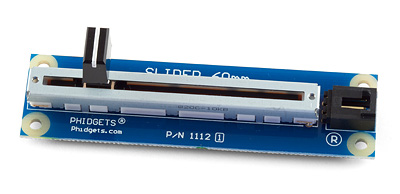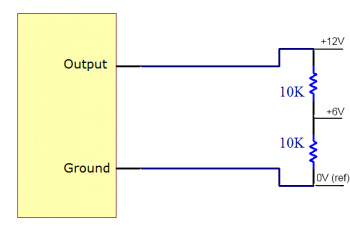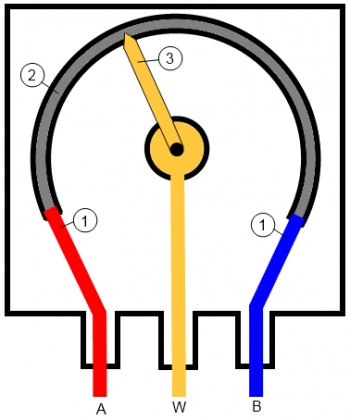# Potentiometer Primer

## IntroductionPotentiometers are three terminal resistors with one contact, known as the wiper, which slides across the device in order to create a variable voltage divider. They can also be used with just 2 terminals connected in which case they behave like a variable resistor or rheostat. Potentiometers are a common choice for human interface devices since they can easily be made to resemble a dial or track slider.

## How Potentiometers Work

### What is a Voltage Divider?

A voltage divider is a network of resistors that is used to reduce the voltage by some factor. The simplest form of voltage divider is a pair of resistors in series. For example, say you have two 10k resistors in series and nothing else connected to a 12V power supply. The following diagrams shows the voltages at various points in the divider:The voltage at the point between the 2 resistors is proportional to Vcc, and the ratio of the resistors. For example if the resistors were 5k and 10k instead of 2 10k then the voltage would be 8V. If the resistors were 10k and 5k, the voltage would be 4V.

### Potentiometer ConstructionThere are 3 pieces to a potentiometer:

1. These are the reference terminals of the potentiometer. You connect Vcc and Gnd to A and B in order to power your voltage divider.
2. This is a resistive material of some kind. It is often graphite though other common materials include resistance wire, carbon particles in plastic, and a ceramic/metal mixture called cermet. In a linear potentiometer, this would be a straight line as opposed to a circular track.
3. The final piece is the wiper. The wiper acts as a third terminal which moves over the resistive section of the device thereby changing the resistance on either side of it. In the case of a rotational potentiometer like the one illustrated above, the wiper is mounted on a pivot point and it can then be adjusted with a knob or screwdriver etc... In a slider style potentiometer the wiper would sit in a track and behave like the sliders on an audio equalizer. As the wiper nears the Vcc terminal, the voltage on the wiper terminal increases. It decreases as the wiper nears the Gnd terminal.

### Taper

The taper of a potentiometer refers to the relationship between the wiper terminal's voltage and the wiper's position. By changing the characteristics of the resistive material the taper can be adjusted. The two most common forms of taper are linear taper and logarithmic taper. In linear taper, the voltage changes linearly with position. Linear tapers are often found in dials on analog equipment, and as feedback devices on linear actuators or servo motors. It then follows that in a logarithmic taper, the voltage varies logarithmically with position. Logarithmic tapers are primarily used in audio equipment since human perception of volume is logarithmic.

### Using a Potentiometer as a Rheostat

One of the nice things about potentiometers is that they can double as a rheostat (a variable resistor). All you need to do is leave one of the reference terminals unconnected. Now you can adjust the resistance between the 2 terminals you are using by moving the wiper. This can be useful if you want to have an adjustable power sink.